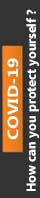We're Open

0% Plagiarism Guaranteed & Custom Written

# Calculate the yield to maturity of a 12-year \$1,000 par value bond with a fixed annual coupon rate of 2.65% and a current price of \$965.40. Provide the solutions for both annual and semi-annual payments of interest.

You are required to set up a separate worksheet (spreadsheet model) for each of the following four problems:

1) Calculate the yield to maturity of a 12-year \$1,000 par value bond with a fixed annual coupon rate of 2.65% and a current price of \$965.40. Provide the solutions for both annual and semi-annual payments of interest. Comment on the relationship between the yield to maturity and the frequency of interest payments, providing an appropriate table or graph.

(20%)

2) Obtain from Bloomberg (or DataStream, or Thomson ONE Banker, or Yahoo Finance) the beginning of month values for the period 1999-2018 of both the IPC MEXICO (^MXX) and the PHLX Semiconductor (^SOX). Assume that you are a risk-averse investor.

For each of the two assets represented by the indices, calculate annual returns and build a frequency distribution of annual returns. Provide graphs of the distributions.

Explore the risk-return relationship for the two assets. Plot your results on a graph with the standard deviation of annual returns of each asset on the horizontal axis and the average annual return on the vertical axis, and comment on which asset performed better.

Assume that you form a portfolio by investing equal amount of money in each asset. Determine the average and standard deviation of the portfolio’s annual returns. Provide your interpretation of the risk and return of the equally-weighted portfolio compared to those of the individual assets.

Calculate and graph the average annual returns and standard deviations of all portfolios that are combinations of the IPC MEXICO (^MXX) and the PHLX Semiconductor (^SOX) with the proportion of the IPC MEXICO (^MXX) being 0, 10, 20, 30, 40, 50, 60, 70, 80, 90, 100%. Comment on the profile of the portfolios.

(35 %) Page 2 of 4

3) For the most recent ten-year period, collect from Bloomberg (or DataStream, or Thomson ONE Banker, or Yahoo Finance) end-of-year values of the NYSE COMPOSITE (DJ) (^NYA) and end-of-year prices of ANY TWO of the member stocks of the NYSE COMPOSITE (DJ) (^NYA) Index. Collect also the latest prices for those two the NYSE COMPOSITE (DJ) (^NYA) stocks.

Assume that the NYSE COMPOSITE (DJ) (^NYA) represents the market portfolio. Compute the excess returns of each of the two stocks that you have selected against that of the NYSE COMPOSITE (DJ) (^NYA). Use the short-term Treasury bill rate as the risk-free rate in the capital asset pricing model (CAPM).

Using the CAPM framework and regression analysis, provide the estimates of both systematic risk and theoretical return for the selected two stocks.

Discuss the results. Explain which of the two stocks has more systematic risk.

(35%)

4) Suppose today is the 20th of May 2019. The January 17th, 2020 call on Facebook Inc. common stock is priced at \$17.50. The exercise price of the call option is \$185.00. The expiry date of the option is January 17th, 2020. The 20th of May 2019 price of Facebook Inc. common stock is \$182.56. The risk free rate is 1.50%.

You are required to derive the implied volatility  (sigma) of the underlying asset from the current market price of January 17th, 2020 call on Facebook Inc. common stock.

Assume a 17% starting value for  to set up a theoretical model of the price of the call option. Assume the stock does not pay a dividend before the expiry date. Comment on the results.

100% Plagiarism Free & Custom Written,International House, 12 Constance Street, London, United Kingdom,
E16 2DQ

## STILL NOT CONVINCED?

We've produced some samples of what you can expect from our Academic Writing Service - these are created by our writers to show you the kind of high-quality work you'll receive. Take a look for yourself!FLAT 25% OFF ON EVERY ORDER.Use "FLAT25" as your promo code during checkout